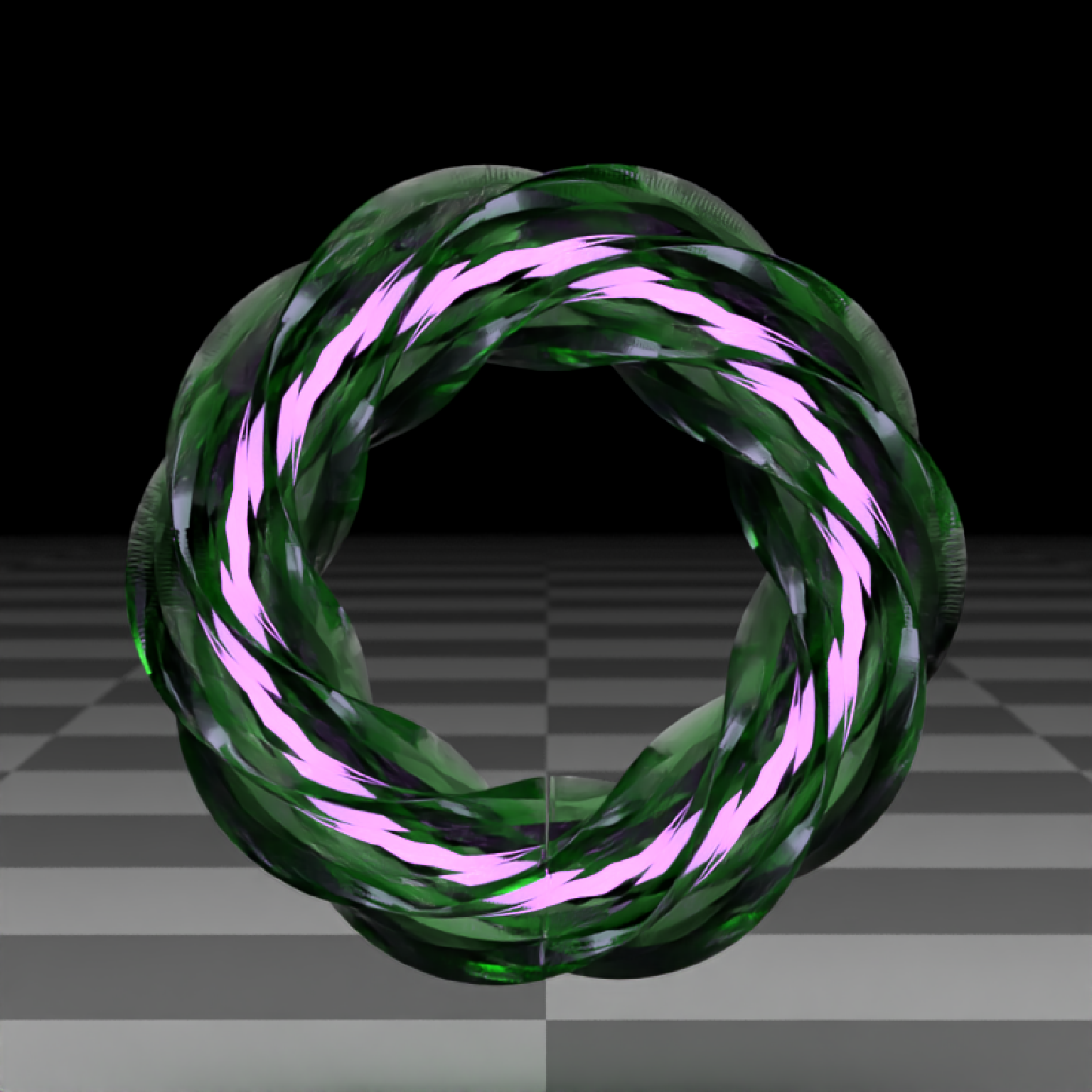Note: Bump mapping with non-diffuse materials does not work correctly, and smoothed normals will be flat when using a bump map.

extruded_path(
points,
x = 0,
y = 0,
z = 0,
polygon = NA,
polygon_end = NA,
breaks = NA,
closed = FALSE,
closed_smooth = TRUE,
twists = 0,
texture_repeats = 1,
straight = FALSE,
precomputed_control_points = FALSE,
width = 1,
width_end = NA,
width_ease = "spline",
smooth_normals = FALSE,
u_min = 0,
u_max = 1,
linear_step = FALSE,
end_caps = c(TRUE, TRUE),
material = diffuse(),
material_caps = NA,
angle = c(0, 0, 0),
order_rotation = c(1, 2, 3),
flipped = FALSE,
scale = c(1, 1, 1)
)

## Arguments

points

Either a list of length-3 numeric vectors or 3-column matrix/data.frame specifying the x/y/z points that the path should go through.

x

Default 0. x-coordinate offset for the path.

y

Default 0. y-coordinate offset for the path.

z

Default 0. z-coordinate offset for the path.

polygon

Defaults to a circle. A polygon with no holes, specified by a data.frame() parsable by xy.coords(). Vertices are taken as sequential rows. If the polygon isn't closed (the last vertex equal to the first), it will be closed automatically.

polygon_end

Defaults to polygon. If specified, the number of vertices should equal the to the number of vertices of the polygon set in polygon. Vertices are taken as sequential rows. If the polygon isn't closed (the last vertex equal to the first), it will be closed automatically.

breaks

Defaults to 20 times the number of control points in the bezier curve.

closed

Default FALSE. If TRUE, the path will be closed by smoothly connecting the first and last points, also ensuring the final polygon is aligned to the first.

closed_smooth

Default TRUE. If closed = TRUE, this will ensure C2 (second derivative) continuity between the ends. If closed = FALSE, the curve will only have C1 (first derivative) continuity between the ends.

Default 0. Positive integer specifying the number of points to fill in between polygon vertices. Higher numbers can give smoother results (especially when combined with smooth_normals = TRUE.

twists

Default 0. Number of twists in the polygon from one end to another.

texture_repeats

Default 1. Number of times to repeat the texture along the length of the path.

straight

Default FALSE. If TRUE, straight lines will be used to connect the points instead of bezier curves.

precomputed_control_points

Default FALSE. If TRUE, points argument will expect a list of control points calculated with the internal rayrender function rayrender:::calculate_control_points().

width

Default 0.1. Curve width. If width_ease == "spline", width is specified in a format that can be read by xy.coords() (with y as the width), and the x coordinate is between 0 and 1, this can also specify the exact positions along the curve for the corresponding width values. If a numeric vector, specifies the different values of the width evenly along the curve. If not a single value, width_end will be ignored.

width_end

Default NA. Width at end of path. Same as width, unless specified. Ignored if multiple width values specified in width.

width_ease

Default spline. Ease function between width values. Other options: linear, quad, cubic, exp.

smooth_normals

Default FALSE. Whether to smooth the normals of the polygon to remove sharp angles.

u_min

Default 0. Minimum parametric coordinate for the path. If closed = TRUE, values greater than one will refer to the beginning of the loop (but the path will be generated as two objects).

u_max

Default 1. Maximum parametric coordinate for the path. If closed = TRUE, values greater than one will refer to the beginning of the loop (but the path will be generated as two objects).

linear_step

Default FALSE. Whether the polygon intervals should be set at linear intervals, rather than intervals based on the underlying bezier curve parameterization.

end_caps

Default c(TRUE, TRUE). Specifies whether to add an end cap to the beginning and end of a path.

material

Default diffuse. The material, called from one of the material functions.

material_caps

Defaults to the same material set in material. Note: emissive objects may not currently function correctly when scaled.

angle

Default c(0, 0, 0). Angle of rotation around the x, y, and z axes, applied in the order specified in order_rotation.

order_rotation

Default c(1, 2, 3). The order to apply the rotations, referring to "x", "y", and "z".

flipped

Default FALSE. Whether to flip the normals.

scale

Default c(1, 1, 1). Scale transformation in the x, y, and z directions. If this is a single value, number, the object will be scaled uniformly.

## Value

Single row of a tibble describing the cube in the scene.

## Examples

if(run_documentation()) {
#Specify the points for the path to travel though and the ground material
points = list(c(0,0,1),c(-0.5,0,-1),c(0,1,-1),c(1,0.5,0),c(0.6,0.3,1))
ground_mat = material=diffuse(color="grey50",
checkercolor = "grey20",checkerperiod = 1.5)
}
if(run_documentation()) {
#Default path shape is a circle
generate_studio(depth=-0.4,material=ground_mat) |>
material=diffuse(color="red"))) |>
render_scene(lookat=c(0.3,0.5,0.5),fov=12, width=800,height=800, clamp_value = 10,
aperture=0.025, samples=128, sample_method="sobol_blue")
}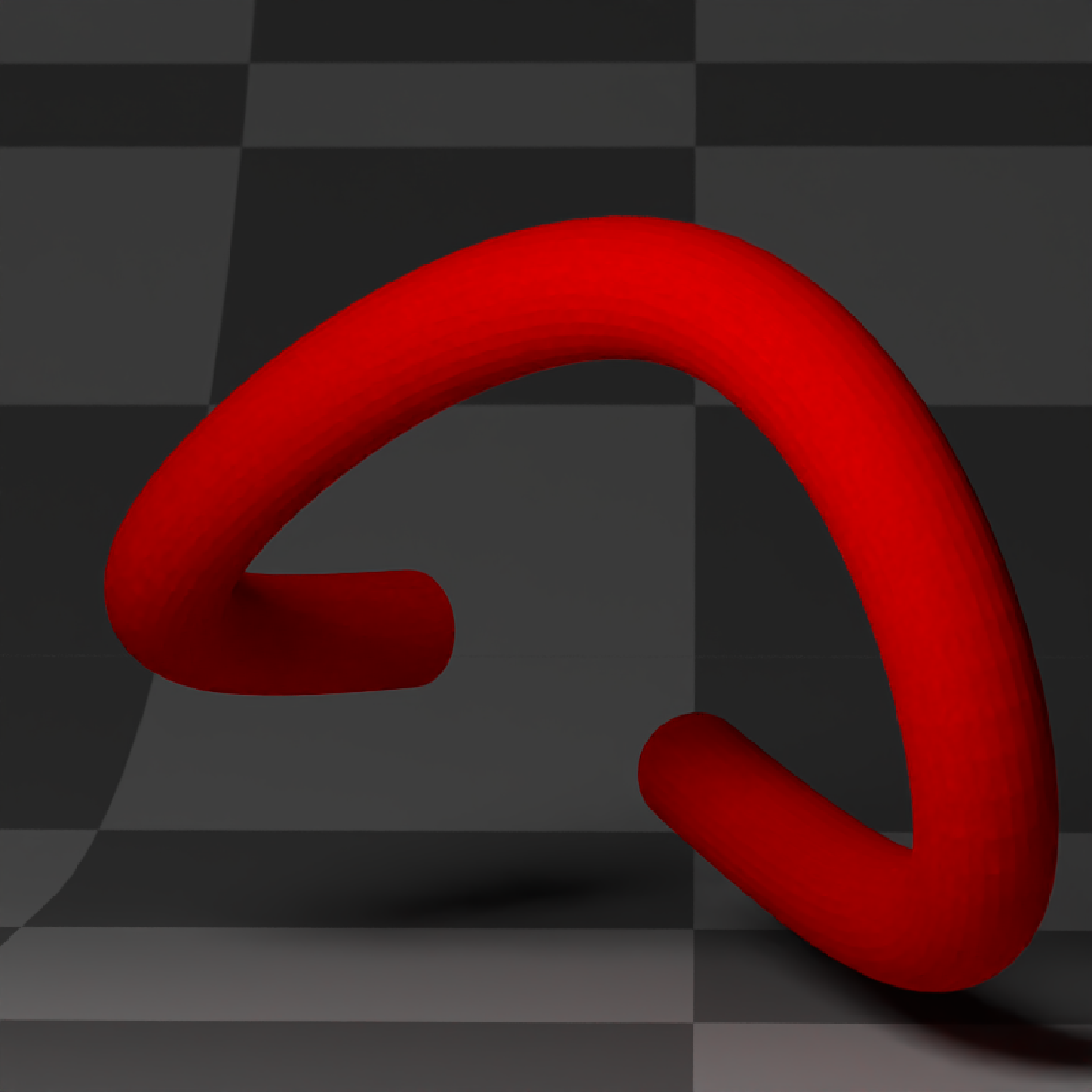if(run_documentation()) {
#Change the width evenly along the tube
generate_studio(depth=-0.4,material=ground_mat) |>
width_end = 0.5,
material=diffuse(color="red"))) |>
render_scene(lookat=c(0.3,0.5,0.5),fov=12, width=800,height=800, clamp_value = 10,
aperture=0.025, samples=128, sample_method="sobol_blue")
}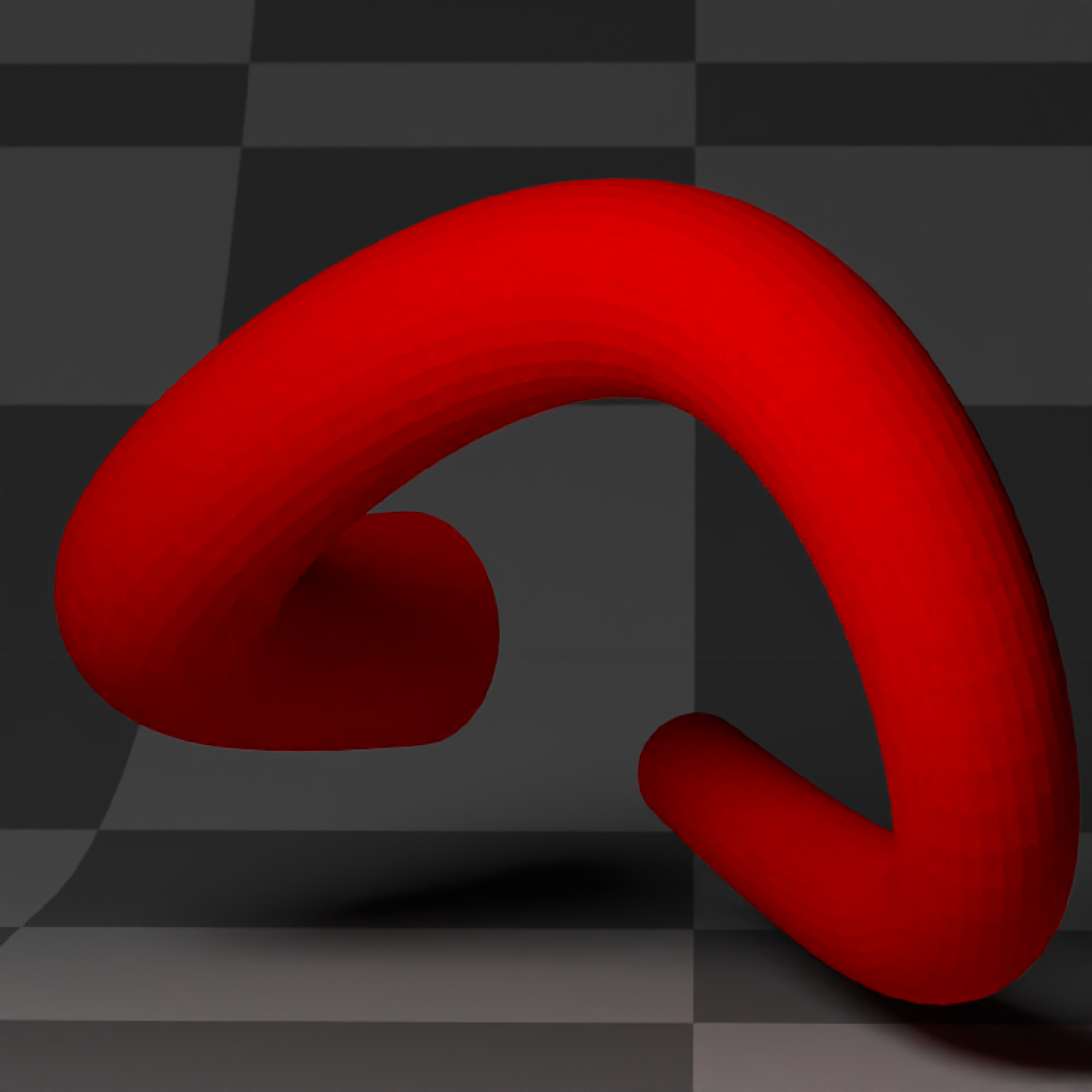if(run_documentation()) {
#Change the width along the full length of the tube
generate_studio(depth=-0.4,material=ground_mat) |>
width=0.25*sinpi(0:72*20/180),
material=diffuse(color="red"))) |>
render_scene(lookat=c(0.3,0.5,0.5),fov=12, width=800,height=800, clamp_value = 10,
aperture=0.025, samples=128, sample_method="sobol_blue")
}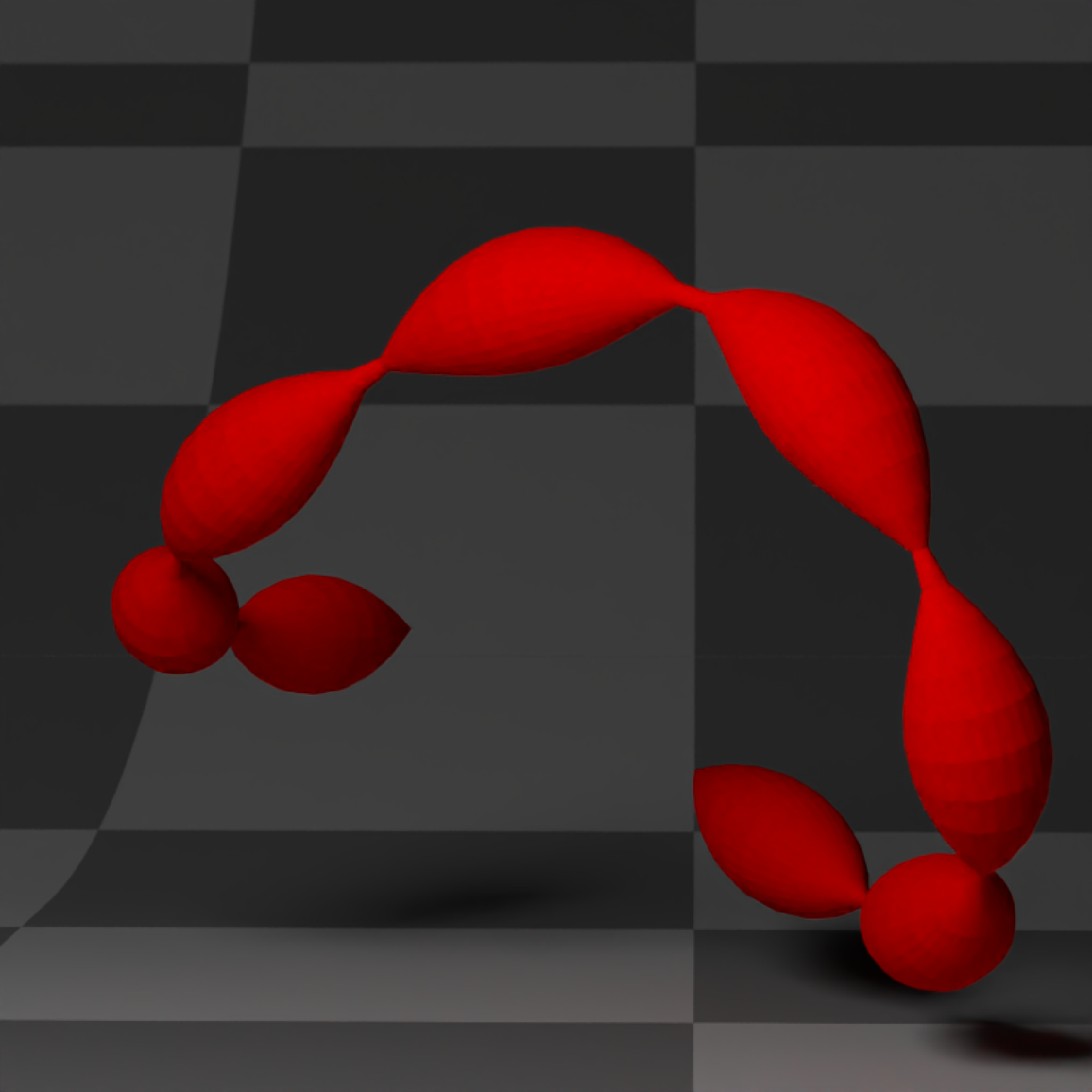if(run_documentation()) {
#Specify the exact parametric x positions for the width values:
custom_width = data.frame(x=c(0,0.2,0.5,0.8,1), y=c(0.25,0.5,0,0.5,0.25))
generate_studio(depth=-0.4,material=ground_mat) |>
width=custom_width,
material=diffuse(color="red"))) |>
render_scene(lookat=c(0.3,0.5,0.5),fov=12, width=800,height=800, clamp_value = 10,
aperture=0.025, samples=128, sample_method="sobol_blue")
}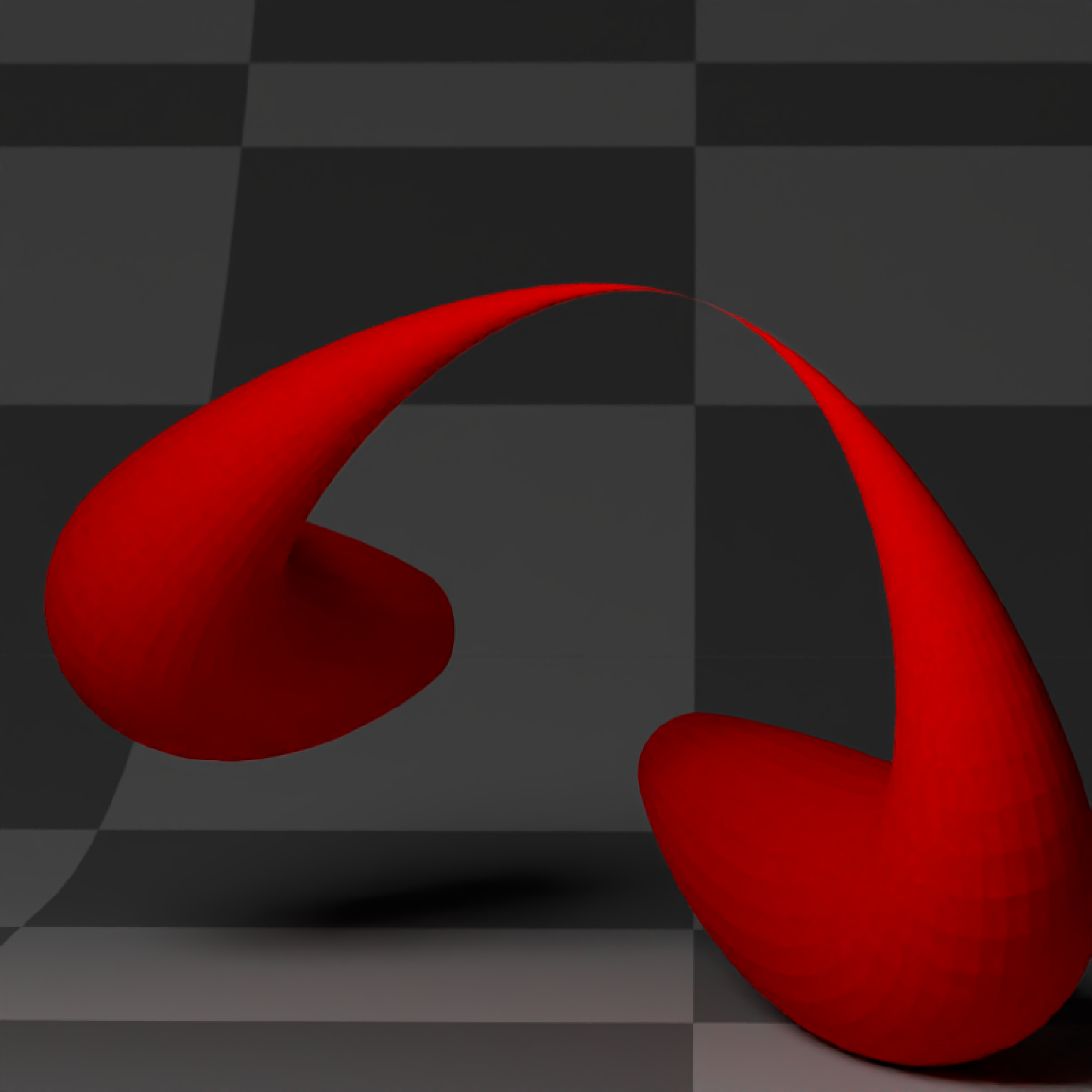if(run_documentation()) {
#Generate a star polygon
angles = seq(360,0,length.out=21)
xx = c(rep(c(1,0.75,0.5,0.75),5),1) * sinpi(angles/180)/4
yy = c(rep(c(1,0.75,0.5,0.75),5),1) * cospi(angles/180)/4
star_polygon = data.frame(x=xx,y=yy)

#Extrude a path using a star polygon
generate_studio(depth=-0.4,material=ground_mat) |>
polygon = star_polygon,
material=diffuse(color="red"))) |>
render_scene(lookat=c(0.3,0.5,1),fov=12, width=800,height=800, clamp_value = 10,
aperture=0.025, samples=128, sample_method="sobol_blue")
}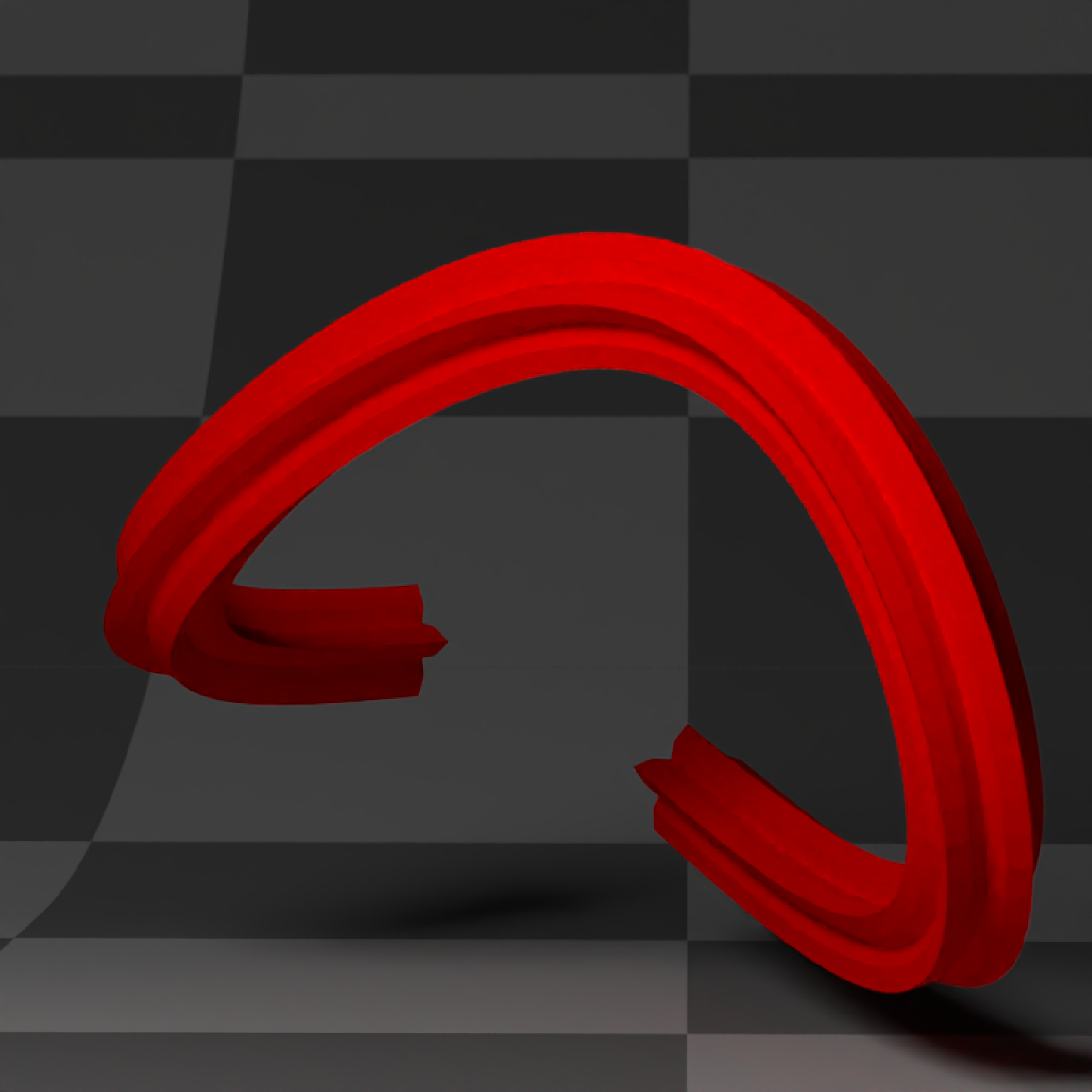if(run_documentation()) {
#Specify a circle polygon
angles = seq(360,0,length.out=21)
xx = sinpi(angles/180)/4
yy = cospi(angles/180)/4
circ_polygon = data.frame(x=xx,y=yy)

#Transform from the circle polygon to the star polygon and change the end cap material
generate_studio(depth=-0.4,material=ground_mat) |>
polygon=circ_polygon, polygon_end = star_polygon,
material_cap  = diffuse(color="white"),
material=diffuse(color="red"))) |>
render_scene(lookat=c(0.3,0.5,0.5),fov=12, width=800,height=800, clamp_value = 10,
aperture=0.025, samples=128, sample_method="sobol_blue")
}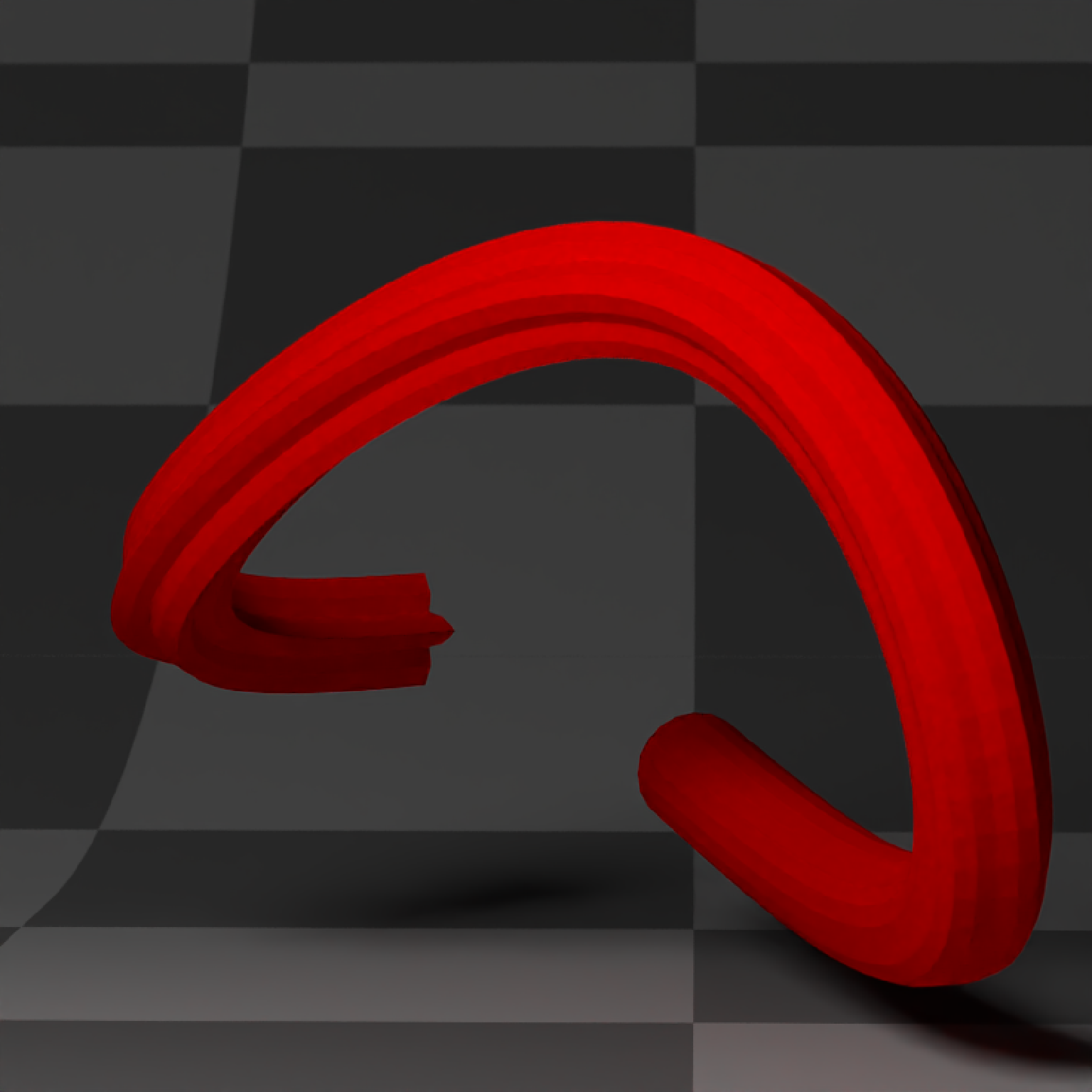if(run_documentation()) {
#Add three and a half twists along the path, and make sure the breaks are evenly spaced
generate_studio(depth=-0.4,material=ground_mat) |>
add_object(extruded_path(points = points, width=0.5, twists = 3.5,
polygon=star_polygon, linear_step = TRUE, breaks=360,
material_cap  = diffuse(color="white"),
material=diffuse(color="red"))) |>
render_scene(lookat=c(0.3,0.5,0),fov=12, width=800,height=800, clamp_value = 10,
aperture=0.025, samples=128, sample_method="sobol_blue")
}if(run_documentation()) {
#Smooth the normals for a less sharp appearance:
generate_studio(depth=-0.4,material=ground_mat) |>
add_object(extruded_path(points = points, width=0.5, twists = 3.5,
polygon=star_polygon,
linear_step = TRUE, breaks=360,
smooth_normals = TRUE,
material_cap  = diffuse(color="white"),
material=diffuse(color="red"))) |>
render_scene(lookat=c(0.3,0.5,0),fov=12, width=800,height=800, clamp_value = 10,
aperture=0.025, samples=128, sample_method="sobol_blue")
}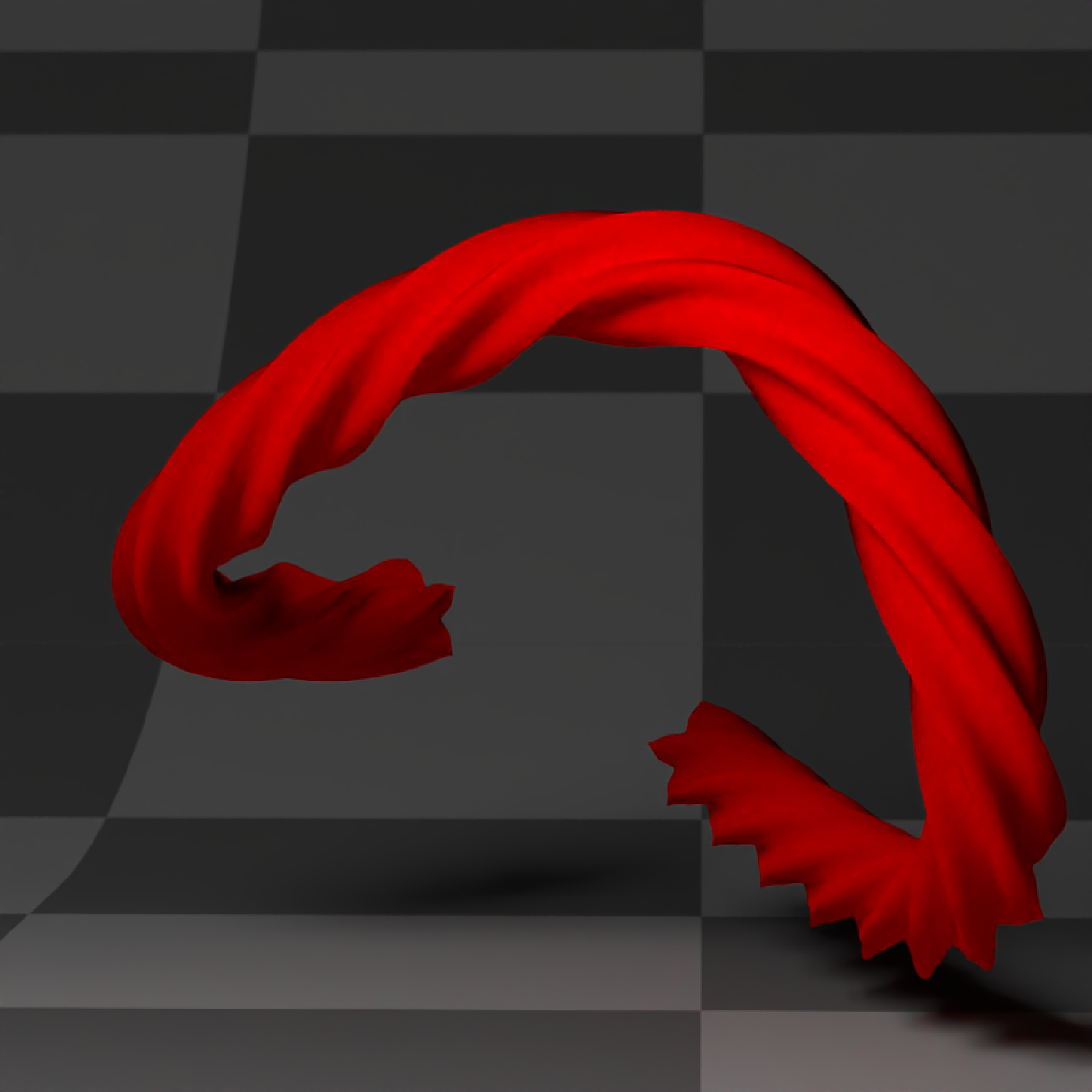if(run_documentation()) {
#Only generate part of the curve, specified by the u_min and u_max arguments
generate_studio(depth=-0.4,material=ground_mat) |>
add_object(extruded_path(points = points, width=0.5, twists = 3.5,
u_min = 0.2, u_max = 0.8,
polygon=star_polygon, linear_step = TRUE, breaks=360,
material_cap  = diffuse(color="white"),
material=diffuse(color="red"))) |>
render_scene(lookat=c(0.3,0.5,0),fov=12, width=800,height=800, clamp_value = 10,
aperture=0.025, samples=128, sample_method="sobol_blue")
}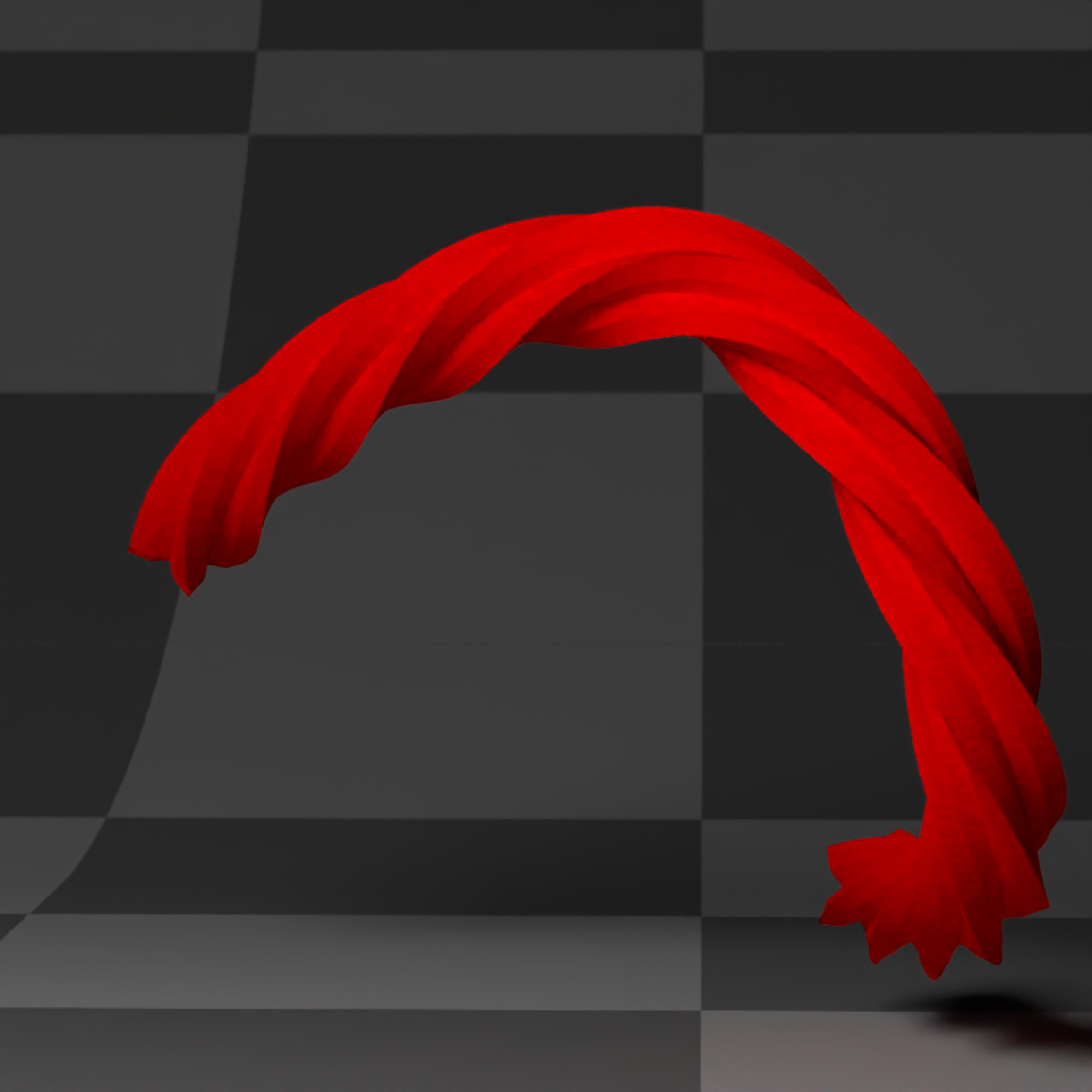if(run_documentation()) {
#Render a Mobius strip with 1.5 turns
points = list(c(0,0,0),c(0.5,0.5,0),c(0,1,0),c(-0.5,0.5,0))
square_polygon = matrix(c(-1, -0.1, 0,
1, -0.1, 0,
1,  0.1, 0,
-1,  0.1, 0)/10, ncol=3,byrow = T)

generate_studio(depth=-0.2,
material=diffuse(color = "dodgerblue4", checkercolor = "#002a61",
checkerperiod = 1)) |>
add_object(extruded_path(points = points,  polygon=square_polygon, closed = TRUE,
linear_step = TRUE, twists = 1.5, breaks = 720,
material = diffuse(noisecolor = "black", noise = 10,
noiseintensity = 10))) |>
render_scene(lookat=c(0,0.5,0), fov=10, samples=128, sample_method = "sobol_blue",
width = 800, height=800)
}if(run_documentation()) {
#Create a green glass tube with the dielectric priority interface
#and fill it with a purple neon tube light
generate_ground(depth=-0.4,material=diffuse(color="grey50",
checkercolor = "grey20",checkerperiod = 1.5)) |>
add_object(extruded_path(points = points, width=0.7, linear_step = TRUE,
polygon = circ_polygon, twists = 2, closed = TRUE,
polygon_end = star_polygon,
material=dielectric(priority = 1, refraction = 1.2,
attenuation=c(1,0.3,1)*10))) |>
add_object(extruded_path(points = points, width=0.4, linear_step = TRUE,
polygon = circ_polygon,twists = 2, closed = TRUE,
polygon_end = star_polygon,
material=dielectric(priority = 0,refraction = 1))) |>
add_object(extruded_path(points = points, width=0.05, closed = TRUE,
material=light(color="purple", intensity = 5,
importance_sample = FALSE))) |>